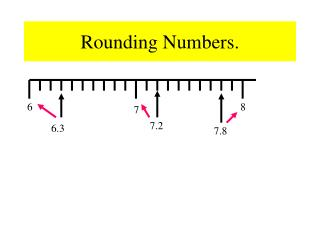DownloadDownload PresentationRounding Numbers.

# Rounding Numbers.

Download Presentation## Rounding Numbers.

- - - - - - - - - - - - - - - - - - - - - - - - - - - E N D - - - - - - - - - - - - - - - - - - - - - - - - - - -
##### Presentation Transcript

1. 7.2 6.3 7.8 6 8 7 Rounding Numbers.

2. 6.5 6.7 6 8 7 Rounding To The Nearest Whole Number. 6.7 Consider the number : It is our task to decide if the number is closer to : 6 7 Look at the number line below: or Mark the number 6.5 6.5 is the midpoint between 6 and 7. Mark the number 6.7 Is 6.7 closer to 6 or 7 ? Is 6.7 above or below 6.5 ? Above 6.7 = 7 (to the nearest whole number)

3. 24.3 24.5 24 26 25 Further Example. Round the following to the nearest whole number: (1) 24.3 What is the midpoint between 24 and 25 ? Is 24.3 above or below 24.5 ? Below. 24.3 = 24 (To the nearest whole number).

4. What Goes In The Box ? Round the following to the nearest whole number: (1) 4.8 5 (6) 287.6 288 (2) 7.4 7 (7) 9.8 10 (3) 44.6 44 (8) 49.6 50 (4) 87.5 88 (9) 99.7 100 (5) 56.3 56 (10) 399.5 400

5. 4.6 4.7 4.65 4.67 4.65 Rounding to the nearest tenth Round 4.67 to the nearest tenth What number is the arrow pointing at ? Mark 4.67 on the number line . Is 4.67 closer to 4.6 or 4.7 ? 4.7 4.67 4.7

6. 19.5 19.6 19.55 19.55 19.53 Round 19.53 to nearest tenth. What number is the arrow pointing at ? Mark 19.53 on the number line . Is 19.53 closer to 19.5 or 19.6 ? 19.5 19.53 19.5

7. What Goes in the Box ? 2 Round the following to nearest tenth. (1) 34.67 = 34.7 (5) 56.95 = 60 (6) 39.97 = 40 (2) 17.72 = 17.7 100 (3) 24.75 = 24.8 (7) 99.96 = (8) 999.95 = 1000 (4) 41.92 = 41.9

8. 7 Round to nearest hundredth In order to make this a faster process we will take a different approach: Round 45.678 to the nearest hundredth What number is in the hundredths place? Is the number after the seven greater or smaller than 5 ? 8 is greater than 5 (or 8 > 5) Rule If the number is greater than or equal to 5 then round up. Now round 45.678 to 45.68 45.678 45.68

9. 8 Round 23.482 to nearest hundredth What number is in the hundredths place? Is the number after the eight greater or smaller than 5 ? 2 is less than 5 (or 2 < 5) Now round 23.482 to the nearest hundredth 23.482 23.48 Rule If the number is greater than or equal to 5 then round up.

10. What Goes in the Box ? Round the numbers below to the nearest hundredth (1) 45.783 = 45.78 (5) 36.995 = 37 (2) 37.677 = 37.68 (6) 99.992 = 99.99 0.1 (3) 6.4589 = 6.46 (7) 0.097 = 0.43 (4) 0.4291 = (8) 999.9958 = 1000

11. What Goes in the Box ? Round the numbers below to the required unit: (1) 34 567 to the nearest ten = 34 570 (2) 47 943 to the nearest hundred = 47 900 357 000 (3) 356 518 to the nearest thousand = (4) 356 218 to the nearest ten thousand = 360 000